# Optimal Fractional-Order PI Control Design for a Variable Speed PMSG-Based Wind Turbine

Optimal Fractional-Order PI Control Design for a Variable Speed PMSG-Based Wind Turbine
Department of Electrical Engineering, University of Tiaret, City of Tiaret 14023, Algeria

Department of Engineering and Technology, University of Hertfordshir, City of Hatfield 19440, United of Kingdom

Corresponding Author Email:
noureddine.sarir@univ-tiaret.dz
Page:
915-922
|
DOI:
https://doi.org/10.18280/jesa.540615
20 August 2021
|
Revised:
10 November 2021
|
Accepted:
19 November 2021
|
Available online:
29 December 2021
| Citation

OPEN ACCESS

Abstract:

This paper focusses on the design of optimal control strategies for a variable-speed wind energy system based on Permanent Magnet Synchronous Generator (PMSG). The fractional order PI controller, denoted PIλ, is an extension of the classical PI controller, which provides greater flexibility, better performance and robustness, however the tuning of the controller parameters is challenging. In this work, Particle Swarm Optimization (PSO) and Genetic Algorithm (GA) provide approximate solutions to various problems and form a good optimization. In our system, they are used to have the PI regulator parameters and tune the parameters of the proposed controllers. The proposed controllers have been applied as maximum power point (MPPT) controllers for the wind turbine and to regulate the PMGS currents under variable weather conditions and. The results show that, among all these controllers, the fractional order PI controller optimized by the PSO leads to better performance in terms of the transient response characteristics such overshoot, rise time and settling time.

Keywords:

wind turbine, MPPT, fractional order PI controller, PSO, GA

1. Introduction

In recent years, the world is experiencing a shift towards the adoption of alternative, cheaper and cleaner energy sources . This is driven by the rapid decline of global reserves of conventional energy resources and the potential threats fossil fuels are causing to the environment such as pollution and global warming . With the global population growth and industrialization on the rise, the demand for electrical energy has witnessed unprecedented level across the globe, which is expected to increase further in the decades ahead.

Nowadays, wind energy is one of the most important renewable energy sources (RESs), which can be used not only as standalone systems to supply electricity to isolated areas but can also be integrated to the utility grid . Wind energy is a pollution-free and inexhaustible source. Therefore, a wind energy generation system could be one of the potential sources of alternative energy for the future . Wind generation systems can be installed close to areas where electricity demand is high for supplying local loads or deployed as wind farms either inland or offshore to supply large power capacity to support the electricity grid.

Wind Energy Conversion Systems (WECSs) generally comprise of a wind turbine, generator, power converter and their controllers . in our project, we are interested in the permanent synchronous generator which is forms an important role as a main component of wind turbine, they are commonly used to convert the mechanical power output of steam turbines, gas turbines, reciprocating engines, and hydro turbines into electrical power for the grid , while Permanent magnet synchronous motors are the most widely used steady-state 3-phase AC motors, which convert electrical energy into mechanical energy. WECS are continuously subjected to various disturbances such as atmospheric conditions which cause variations in the wind speed. WECS are generally designed to extract maximum power from the wind under different conditions by controlling the current and voltage of the wind generator [7-9]. Several control schemes have been proposed in the literature to improve the performance of the generator and enhance the efficiency of WECS. Proportional-Integral (PI) controllers are simple to implement and can achieve a good transient and steady state response with small overshoot and short settling-time and have been widely used in industrial processes [10, 11]. However, these classical controllers may not be very effective for systems exhibiting nonlinearities and parameter variations.

Many real-world physical systems are modeled by fractional-order differential equations, that is, equations involving non integer-order integrals such as dynamic systems characterized by memory and hereditary effects . In recent years, fractional calculus has been applied to the modeling and control of various types of physical systems, and this is well documented in many control theories and applications literature. The concept of fractional-order control has been used to design PI like controllers with enhanced robustness and improved performance than the classical PI controllers.

The so-called Fractional-Order PI controller (FOPI), besides the proportional (Kp) and integral (Ki) gains, has an additional parameter termed the fractional integration order denoted by λ. Therefore, the FOPI has three tuning parameters which make it more flexible . There are several powerful optimization methods which can be employed to determine the optimal set of parameters for the FOPI. In this paper, the PSO (Particle Swarm Optimization) and GA (Genetic Algorithm) methods are used to optimize the parameters of the classical PI and FOPI controllers [14-16]. the new task in our work is to make limits to limit the search for optimization point in this interval, this method will help us to choose the most efficient method between the PSO and GA.

The remaining of the paper is organized in following flowchart:

## 1.png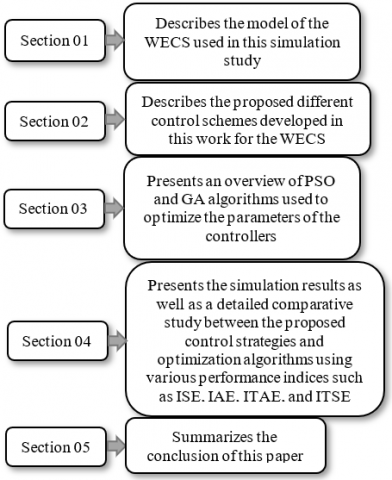2. Modeling of the Wind Energy Conversion System

In Figure 1 is depicted the WECS used in this study.

## 00.png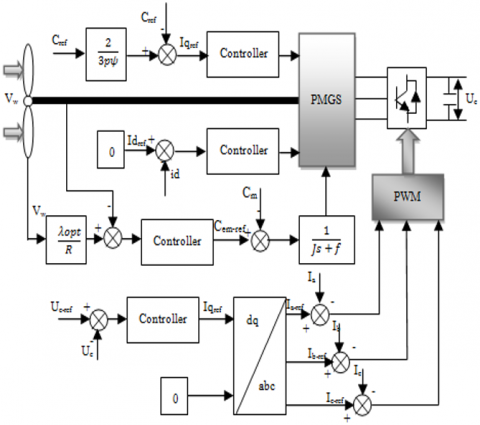Figure 1. Configuration of the WECS and its control scheme

2.1 Wind turbine model

The maximum power generated by the wind turbine is expressed as :

${{P}_{m}}=\frac{1}{2}.\rho .S.{{v}^{3}}.{{C}_{p}}(\lambda ,\beta )$      (1)

where, ρ is the density is the density of the air, S is the area swept by the blades of the turbine; λ is tip-speed ratio and β is the blade pitch angle, v is the wind speed in meters per second and Cp is the power coefficient .

The mechanical torque Cm seen by the synchronous machine is different from the wind torque Cem because it is necessary to consider the inertia of the wind turbine and the viscous friction specific to the structure of the wind turbine. This can be mathematically translated by the following equation :

${{C}_{em}}=J\frac{d\Omega }{dt}+f\Omega +{{C}_{m}}$     (2)

$\Omega =\frac{1}{Js+f}({{C}_{em}}-{{C}_{m}})$       (3)

where, J and f represent the inertia of the wind turbine and the friction of the wind turbine respectively, s denotes Laplace operator. Figure 2 shows the bloc diagram representing the dynamic model of the wind turbine.

## 22.png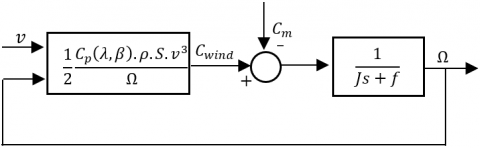Figure 2. Dynamic model of the wind turbine

2.2 Permanent magnet synchronous generator model

## 3.png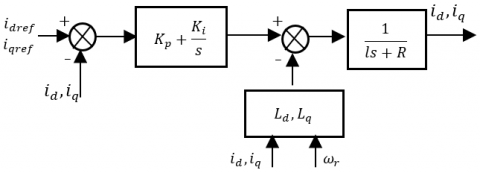Figure 3. Currents id, iq control loop

The mathematical model of the PMSG in the synchronous reference frame (in the state equation form) is given by Eq. (4) [20, 21]:

$\left\{ \begin{matrix} \frac{d{{i}_{d}}}{dt}=\frac{1}{{{l}_{d}}}(-R{{i}_{d}}+{{\omega }_{e}}{{l}_{q}}{{i}_{q}}+{{V}_{d}}) \\ \frac{d{{i}_{q}}}{dt}=\frac{1}{{{l}_{q}}}(-R{{i}_{q}}+{{\omega }_{e}}{{l}_{d}}{{i}_{d}}-{{\phi }_{f}}{{\omega }_{e}}+{{V}_{q}}) \\ {{C}_{em}}=\frac{3}{2}P({{l}_{q}}-{{l}_{d}}){{i}_{d}}{{i}_{q}}+{{i}_{q}}{{\phi }_{f}} \\\end{matrix} \right.$       (4)

where, R is the stator resistance, ϕf is the permanent magnetic flux, and Vd, Vq, id, iq, ld and lq are the d and q-component of the stator voltage, current, and inductance, respectively and P is number of pole pairs. The machine can be represented by the following transfer function :

${{G}_{s}}(s)=\frac{1}{ls+R}=\frac{{{i}_{d,q}}(s)}{{{V}_{d,q}}(s)+{{E}_{d,q}}(s)}$      (5)

where, $E_{d, q}=\pm \omega_{e} \phi_{f}$ are decoupling terms and considered as perturbations. The control loops of the current id and iq are shown in Figure 3 by the block diagram.

3. Fractional PI Controller

In fractional-order calculus, the fundamental differ-integration operator $a D_{t}^{\alpha}$ (where a and t are the limits of the operation) is defined as :

$aD_{t}^{\alpha }=\left\{ \begin{matrix} \begin{matrix} \frac{{{d}^{\alpha }}}{dt} & \alpha >0 \\\end{matrix} \\ \begin{matrix} 1 & \alpha =0 \\\end{matrix} \\ \begin{matrix} \int\limits_{a}^{t}{d{{t}^{\alpha }}} & \alpha <0 \\\end{matrix} \\\end{matrix} \right.$       (6)

where, α is the order of the operation, generally αR but α could also be a complex number. Fractional calculus has a larger range than integer order derivative. If a factional order is used instead of the integer order in the derivative or integral, then a fractional calculus must be used to solve this fractional derivative and integral . The generalized fractional differentiation and integration has mainly three definitions, the Grunwald-Letnikov (G-L) definition and the Riemann-Liouville (R-L) definition and the Caputo definition .

Grunwald-Letnikov’s definition is perhaps the best known one due to its suitability for the design of discrete control algorithms. The m order fractional derivative of a continuous function f(t) is given by :

$aD_{t}^{\alpha }f(t)=\underset{h\to 0}{\mathop{\lim }}\,\frac{1}{{{h}^{\alpha }}}\sum\nolimits_{j=0}^{(\tfrac{t-a}{h})}{{{(-1)}^{j}}\left( \begin{matrix} \alpha \\ j \\\end{matrix} \right)f(t-jh)}$      (7)

In Eq. (7), $\left[\frac{(t-a)}{h}\right]$ truncates $\frac{(t-a)}{h}$ to an integer. To determine a fractional-order transfer function, consider a linear time-invariant fractional model of a system with input u and output y takes the following form :

\begin{align} {{a}_{n}}{{D}^{{{\lambda }_{n}}}}y(t)+{{a}_{n-1}}{{D}^{{{\lambda }_{n-1}}}}y(t)+...+{{a}_{0}}{{D}^{{{\lambda }_{0}}}}y(t)= & \\ {{b}_{m}}{{D}^{{{\mu }_{m}}}}u(t)+{{b}_{m-1}}{{D}^{{{\mu }_{m-1}}}}u(t)+...+{{b}_{0}}{{D}^{{{\mu }_{0}}}}u(t) & \\\end{align}       (8)

where, ai, λi (i = 0, 1, ..., n), bk, µk (k = 0, 1, ..., m), are real constants, n and m are positive integers. The Laplace transform of the fractional derivative of f(t) for zero conditions given by:

$L(aD_{t}^{\alpha }f(t))={{S}^{\alpha }}f(t)$   (9)

Therefore, the Laplace transform on both sides of Eq. (10) for zero initial conditions leads to the following transfer function:

$\frac{Y(s)}{U(s)}=\frac{{{b}_{m}}{{D}^{{{\mu }_{m}}}}+{{b}_{m-1}}{{D}^{{{\mu }_{m-1}}}}+...+{{b}_{0}}{{D}^{{{\mu }_{0}}}}}{{{a}_{n}}{{D}^{{{\lambda }_{n}}}}+{{a}_{n-1}}{{D}^{{{\lambda }_{n-1}}}}+...+{{a}_{0}}{{D}^{{{\lambda }_{0}}}}}$      (10)

These concepts can be employed to design fractional order controllers such as fractional order PI controller (FOPI). FOPI exhibits excellent performance and robustness and has been successfully employed in several applications. FOPI has an additional tuning parameter as compared to the classical PI controller which provides more flexibility in the design . The continuous transfer function of FOPI is obtained through Laplace transform is given by:

${{G}_{s}}(s)={{K}_{p}}+{{K}_{i}}{{s}^{\lambda }}$      (11)

4. Optimization of PI and FOPI Parameters Using PSO and GA Algorithms

The selection of appropriate gains, Kp, Ki for the PI controller and Kp, Ki, λ for the FOPI controller which is tuned according to the system specifications and which satisfies the robustness of closed loop performance over a wide frequency range is the main objective of the PI controller optimizer. Figure 5 shows the structure of the PI and FOPI controllers optimization process. PSO and GA are used to adjust parameters of PI and FOPI controllers repeatedly until they reach a satisfactory performance level.

## 4.png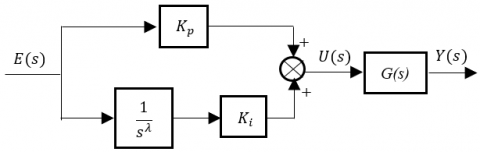Figure 4. Block diagram of fractional order PI controller (PIλ)

## 5.png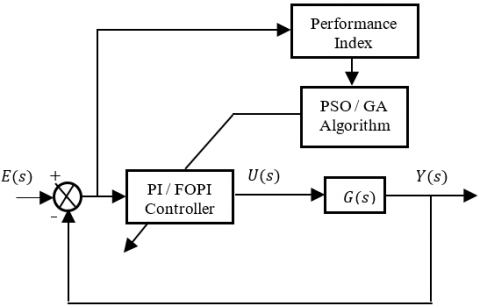Figure 5. Block diagram of PI and FOPI tuning

G(s) represents the transfer function of the turbine G1(s) and the PMSG G2(s), they are given from Figure 2 and Figure 4 respectively by:

$\left\{ \begin{matrix} {{G}_{1}}(s)=\frac{1}{Js+f} \\ {{G}_{2}}(s)=\frac{1}{ls+R} \\\end{matrix} \right.$     (12)

4.1 Overview of heuristic optimization

4.1.1 Particle Swarm Optimization (PSO)

Particle Swarm Optimization (PSO) technique, proposed by Kennedy and Eberhart in 1995 is an evolutionary-type global optimization technique developed due to the inspiration of social activities in flock of birds and school of fish and is widely applied in various engineering problems due to its high computational efficiency. It has been proven that PSO is simple and robust against nonlinearity, non-differentiability and high dimensionality.

The advantages of PSO compared to other optimization techniques are as follow: easy implementation, few parameters to be adjusted and no gradient information is required . Each particle generated by the PSO algorithm has the potential to be the best solution for the problem. At the beginning, each particle of the population is distributed randomly through the search space. The velocity and position are updated by the following two best values. The best value achieved for each particle is the best solution which is called personal best (pbest) and the best value achieved so far among all particles is called 6 global best (gbest). The modified velocity of each particle can be calculated via the present velocity and distance form (pbest) and (gbest) as shown in following equation:

$V_{i}^{t+1}=wV_{i}^{t}+{{c}_{1}}{{R}_{1}}(p_{pbest}^{t}-X_{i}^{t})+{{c}_{2}}{{R}_{2}}(g_{gbest}^{t}-X_{i}^{t})$     (13)

The new position is then determined by the sum of the previous position and the new velocity:

$X_{i}^{t+1}=X_{i}^{t}+V_{i}^{t}$      (14)

where, c1 and c2 are acceleration constants, R1 and R2 represent random values in the range [0 1], $V_{i}^{t}$ and $X_{i}^{t}$ denote the velocity and position of the ith particle at iteration t, w is inertia weight factor, (pbest) are the best position for each particle, (gbest) are the global best value of the group. A detailed flowchart of PSO considering the above steps is shown in Figure 6.

## 6.png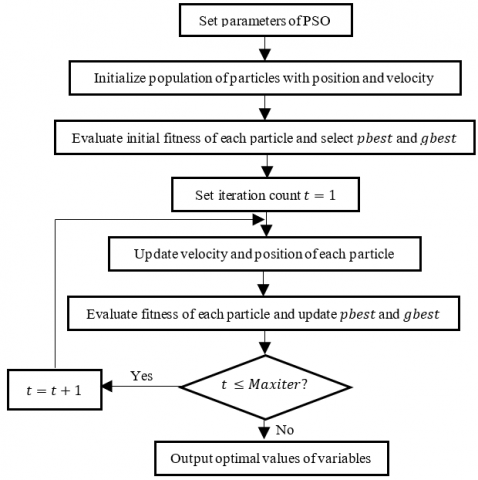Figure 6. Flowchart of PSO

4.1.2 Genetic Algorithm (GA)

Genetic Algorithm (GA) is a stochastic optimization algorithm that mimics the process of natural evolution and which are developed based on the Darwinian theory of “survival of fittest”. GA has three basic operators: selection, crossover and mutation. It has been recognized as an effective and efficient technique to solve optimization problems . Figure 7 shows flowchart of GA.

## 7.png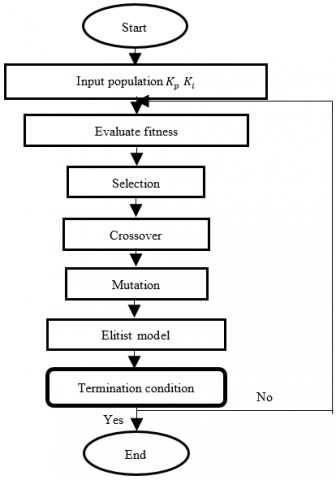Figure 7. Flowchart of GA

We can cite the advantages and disadvantages of PSO and GA algorithms in the following Table 1:

 Advantages disadvantages - They are easy to implements and there are few parameters to adjust. - have already been a new and fast developing research topic -Despite the simplicity and ease of use of the PSO and GA algorithms it presents a risk of divergence or rapid convergence which allows to stagnate in a local optimum, therefore several improvements have been made to the base algorithm

4.2 Performance indices

Table 2. Performance indices

 Performance index Definition Integral of Square Error (ISE) $\int_{0}^{t s s} e^{2}(t) d t$ Integral of Absolute Error (IAE) $\int_{0}^{t s s}|e(t)| d t$ Integral of Time Absolute Error (ITAE) $\int_{0}^{t s s} t|e(t)| d t$ Integral of Time Square Error (ITSE) $\int_{0}^{t s s} t e^{2}(t) d t$

The performance index is defined as a quantitative measure to describe the performance of the designed PI controller system. The goal of optimization based on PSO and GA is to find a set of parameters Kp, Ki and λ such that the feedback control system that minimizes the performance index . There are four commonly used indices to describe system performance are listed in Table 2 . tss is total simulation time and we his tacked 01 second with a step tss=0.01second.

For the fractional PI and PIλ tuning based on PSO and GA, the ITAE performance index produced the best result over the other indices and it will be used as an objective function. Note: interval chosen to determine the best performance index among the four is ranges from [0 0] to [1000 1000].

5. Simulation Results and Discussion

The PSO and GA parameters used in the simulation are given in Tables 3 and 4 in the Appendix. PSO and GA optimization algorithms have been tested using the performance indices given Table 2, and the results are summarized in Tables 5 and 6.

Table 3. PSO parameters

 Parameters Values Population size 49 Number of iterations 50 Number of variables 2(Kp,Ki) and 3(Kp, Ki ,λ ) Inertia weight w 0.9 Acceleration constant c1, c2 2 Range of variables (Kp, Ki, λ) [200 200] and [200 200 1]

Table 4. GA parameters

 Parameters Values Population size 50 Number of variables 2(Kp,Ki) and 3(Kp, Ki ,λ ) Range of variables (Kp, Ki, λ) [200 200] and [200 200 1] Generation 100 Crossover Single point Mutation 0.01 Encoding Binary

Table 5. PI parameters optimization for transfer function G1(s)

 Tuning method Kp Ki Best fitness iteration GA-PI1 (ISE) 3.494 900.554 -0.4707 73 GA-PI2 (IAE) 979.423 998.552 0.0118 51 GA-PI3 (ITAE) 1.85 957.365 8.1873e-7 51 GA-PI4 (ITSE) 1.157 524.559 -0.4961 54

Table 6. PI parameters optimization for transfer function G2(s)

 Tuning method Kp Ki Best fitness iteration PSO-PI1 (ISE) 0 673.2058 -0.8300 73 PSO-PI2 (IAE) 1000 1000 0.0118 51 PSO-PI3 (ITAE) 0 662.5512 9.3902e-06 51 PSO-PI4 (ITSE) 0 847.1597 -0.4872 54

From Figures 8 and 9, it can be seen that the ITAE performance index gives better optimization results compared to other indices and it will subsequently used in the rest of the paper for the comparison of the proposed controllers. A detailed comparative study between PI and FOPI optimized by PSO, and PI and FOPI optimized by GA is performed to determine best control approach for our wind energy system. The results are presented in Figures 10-13.

## 8.png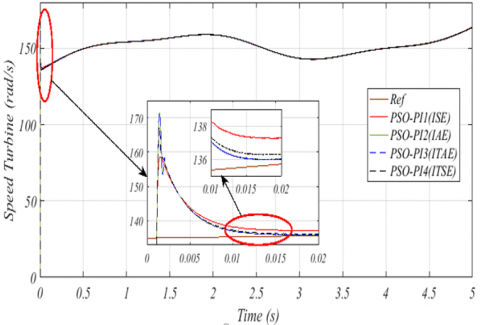Figure 8. Speed turbine response for ISE, IAE, ITAE and ITSE using PSO

## 9.png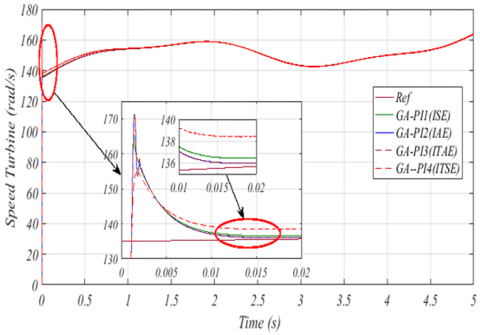Figure 9. Speed turbine response for ISE, IAE, ITAE and ITSE using GA

Note: the controllers are applied to adjust the currents id and iq of the PMGS.

## 10.png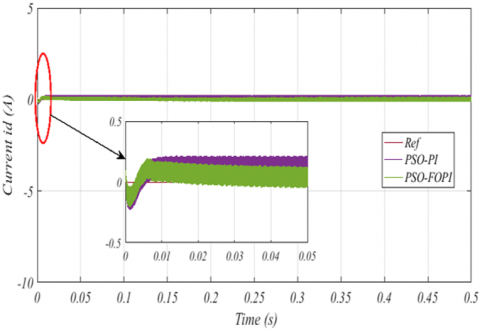Figure 10. Response of the current id with PI and FOPI optimized using PSO

## 11.png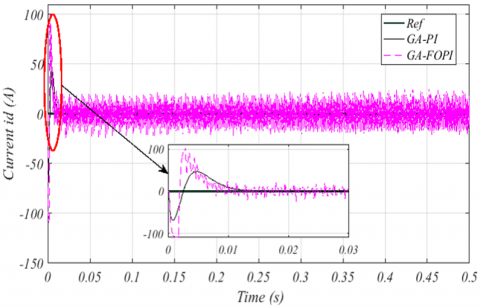Figure 11. Response of the current id with PI and FOPI optimized using GA

## 12.png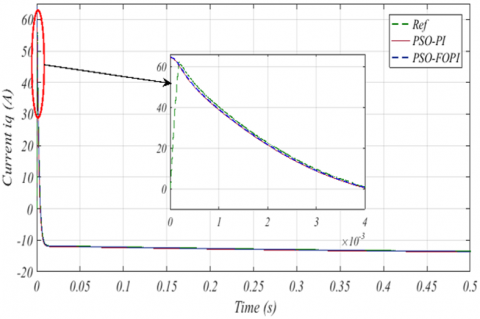Figure 12. Response of the current iq with PI and FOPI optimized using PSO

## 13.png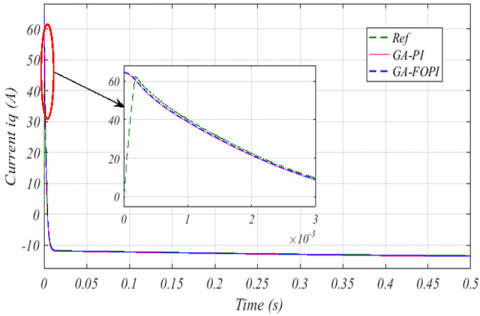Figure 13. Response of the current iq with PI and FOPI optimized using GA

From Figures 10, 11, 12 and 13, it can be noticed that FOPI optimized by the PSO gives better performance compared to the PI optimized by the PSO while FOPI optimized by GA leads to a poor performance compared to the optimized PI by GA.

Finally, a comparative study between PSO-FOPI and GA-PI is undertaken to determine the best controller among all the proposed controllers. The results are presented in Figures 14 and 15. To confirm all the results of the simulation for all figures, Tables 7 and 8 shows the parameters of PI and FOPI regulators and performance of the two algorithms PSO and GA.

## 14.png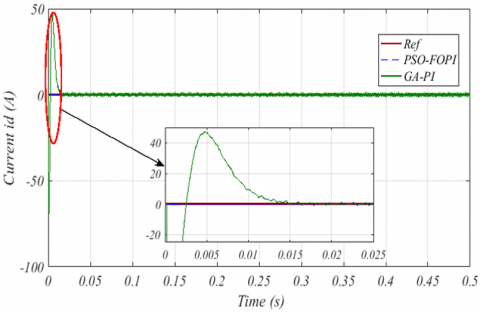Figure 14. Response of the current id with PI and FOPI optimized using PSO and GA

## 15.png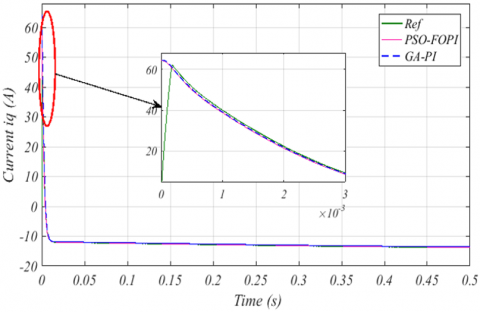Figure 15. Response of the current iq with PI and FOPI optimized using PSO and GA

6. Conclusion

This paper proposed the control of a wind energy conversion system using PI and fractional-order PI (FOPI) optimized using generic algorithm (GA) and particle swarm optimization (PSO) techniques. Four controllers have been evaluated with different performance indices ITAE, IAE, ISE and ITSE for the optimal tuning of the maximum power point (MPPT) of the wind turbine and the regulation of the currents of the permanent magnet synchronous generator (PMSG). The ITAE method gave better results. The designed controllers have been compared based on a set of time domain and frequency domain criteria including overshoot, rise time and settling time. The results obtained have showed that the fractional order PI controller optimized by the PSO leads to better performance in terms of the transient response characteristics. For example, the error bar of GA for FOPI is 0.00356 while the error of PSO for FOPI is 3.0789e-04. The Tables 7, 8 and Figures 14, 15 show the differences between the two algorithms.

Appendix

Tables 7 and 8 show a comparative study in terms of the dynamic and robustness of the PSO and GA controls for both PI and FOPI regulators. The +, - and 0 signs are used to measure the performance of each command.

++: very good performance

+: good performance

0: average performance

-: poor performance

--: very poor performance

Table 7. Comparison between PSO and GA methods for PI controller

 Methods Transfer function Dynamic Robustness Rapidity Kp Ki Best fitness PSO G1(s) + + + 133.6583 167.2180 1.4855e-09 G2(s) + + + 200 200 0.0038 GA G1(s) 0 0 0 115.413 144.266 7.3066e-15 G2(s) 0 0 0 133.6583 167.2180 1.5512e-4

Table 8. Comparison between PSO and GA methods for FOPI controller

 Methods Transfer function Dynamic Robustness Rapidity Kp Ki λ Best fitness PSO G1(s) ++ ++ ++ 159.9851 200 1 5.0163e-08 G2(s) ++ ++ ++ 0 200 1 3.0789e-04 GA G1(s) + + + 147.346 184.191 1 5.4366e-8 G2(s) + + + 200 200 0.394 0.00356
References

 Dursun, E.H., Koyuncu, H., Kulaksiz, A.A. (2021). A novel unified maximum power extraction framework for PMSG based WECS using chaotic particle swarm optimization derivatives. Engineering Science and Technology, an International Journal, 24(1): 158-170. https://doi.org/10.1016/j.jestch.2020.05.005

 Hannachi, M., Elbeji, O., Benhamed, M., Sbita, L. (2019). Maximum power point tracking of a PMSG wind turbine with On-Off control based particle swarm optimization. In 2019 International Conference on Signal, Control and Communication (SCC), pp. 76-80. https://doi.org/10.1109/SCC47175.2019.9116142

 Hamouda, N., Benalla, H., Hemsas, K., Babes, B., Petzoldt, J., Ellinger, T., Hamouda, C. (2017). Type-2 fuzzy logic predictive control of a grid connected wind power systems with integrated active power filter capabilities. Journal of Power Electronics, 17(6): 1587-1599. https://doi.org/10.6113/JPE.2017.17.6.1587

 Das, K.K., Buragohain, M. (2015). An algorithmic approach for maximum power point tracking of wind turbine using particle swarm optimization. Int. J. Adv. Res. Electric. Electron. Instrum. Eng, 4(5): 4099-4106.

 Dalala, Z.M., Zahid, Z.U., Yu, W., Cho, Y., Lai, J.S. (2013). Design and analysis of an MPPT technique for small-scale wind energy conversion systems. IEEE Transactions on Energy Conversion, 28(3): 756-767. https://doi.org/10.1109/TEC.2013.2259627

 Hamied, M.A.A.E., Amary, N.H.E. (2016). Permanent magnet synchronous generator stability analysis and control. Procedia Computer Science, 95: 507-515. https://doi.org/10.1016/j.procs.2016.09.328

 Maroufi, O., Choucha, A., Chaib, L. (2020). Hybrid fractional fuzzy PID design for MPPT-pitch control of wind turbine-based bat algorithm. Electrical Engineering, 102: 2149-2160. https://doi.org/10.1007/s00202-020-01007-5

 Qais, M.H., Hasanien, H.M., Alghuwainem, S. (2018). A grey wolf optimizer for optimum parameters of multiple PI controllers of a grid-connected PMSG driven by variable speed wind turbine. IEEE Access, 6: 44120-44128. https://doi.org/10.1109/ACCESS.2018.2864303

 Fathy, R.M., Ebrahim, M.A., Bendary, F.M. (2016). Automatic tuning of (PID) and fuzzy controllers using (PSO) algorithm for hybrid energy systems. International Journal of Scientific and Engineering Research, 7(3).

 Myrtellari, A., Marango, P., Gjonaj, M. (2015). Optimal control of DC motors using PSO algorithm for tuning PID controller. International Journal of Business and Technology, 4: 8.

 Momani, S., El-Khazali, R., Batiha, I.M. (2019). Tuning PID and PIλDµ Controllers Using Particle Swarm Optimization Algorithm via El-Khazali’s Approach. AIP Conference Proceedings, Ajman University.

 Ahuja, A., Aggarwal, S.K. (2014). Design of fractional order PID controller for DC motor using evolutionary optimization techniques. WSEAS Transactions on System and Control, 9: 171-182.

 Anguluri, R., Abraham, A., Snasel, V. (2011). A Hybrid Bacterial foraging-PSO algorithm based tuning of optimal FOPI speed controller. Acta Montanistica Slovaca, 16(1): 55.

 Mohamed, M.J., Khashan, A. (2014). Comparison Between PID and FOPID Controllers Based on Particle Swarm Optimization. In The Second Engineering Conference of Control, Computers and Mechatronics Engineering ECCCM2.

 Khalfa, B., Abdelfateh, C. (2017). Optimal tuning of fractional order Pλ D µA controller using Particle Swarm Optimization algorithm. IFAC-PapersOnLine, 50(1): 8084-8089. https://doi.org/10.1016/j.ifacol.2017.08.1241

 Bingül, Z.A.F.E.R., Karahan, O.Ğ.U.Z.H.A.N. (2012). Fractional PID controllers tuned by evolutionary algorithms for robot trajectory control. Turkish Journal of Electrical Engineering & Computer Sciences, 20: 1123-1136. http://dx.doi.org/10.3906/elk-1102-1011

 Putri, R.I., Pujiantara, M., Priyadi, A., Hery, P.M. (2016). Optimum control strategy of grid connected PMSG wind turbine based on energy storage system. In 2016 International Seminar on Intelligent Technology and Its Applications (ISITIA), pp. 623-628. https://doi.org/10.1109/ISITIA.2016.7828732

 Ananth, D.V.N., Kumar, G.V.N. (2016). Tip speed ratio based MPPT algorithm and improved field oriented control for extracting optimal real power and independent reactive power control for grid connected doubly fed induction generator. International Journal of Electrical and Computer Engineering, 6(3): 1319. https://doi.org/10.11591/ijece.v6i3.9306

 Zaimi, A. (2014). Optimisation de la commande d’une éolienne à base de machine synchrone à aimants permanents. mémoire en vue d'obtenir le diplôme d'ingénieur CNAM, conservatoire national des arts et métiers centre du Maroc.

 Rolan, A., Luna, A., Vazquez, G., Aguilar, D., Azevedo, G. (2009). Modeling of a variable speed wind turbine with a permanent magnet synchronous generator. In 2009 IEEE International Symposium on Industrial Electronics, pp. 734-739. https://doi.org/10.1109/ISIE.2009.5218120

 Kim, Y.S., Chung, I.Y., Moon, S.I. (2015). Tuning of the PI controller parameters of a PMSG wind turbine to improve control performance under various wind speeds. Energies, 8(2): 1406-1425. https://doi.org/10.3390/en8021406

 Hamecha, S. (2013). Etude et commande d'une éolienne à base d'une machine synchrone à aimants permanents (Doctoral dissertation, Université Mouloud Mammeri).

 Bhookya, J., Jatoth, R.K. (2019). Optimal FOPID/PID controller parameters tuning for the AVR system based on sine–cosine-algorithm. Evolutionary Intelligence, 12(4): 725-733. https://doi.org/10.1007/s12065-019-00290-x

 Faieghi, M.R., Nemati, A. (2011). On fractional-order PID design. In Applications of MATLAB in Science and Engineering. IntechOpen. https://doi.org/10.5772/22657

 Pan, I., Das, S. (2012). Chaotic multi-objective optimization based design of fractional order PIλDμ controller in AVR system. International Journal of Electrical Power & Energy Systems, 43(1): 393-407. https://doi.org/10.1016/j.ijepes.2012.06.034

 Cao, J.Y., Cao, B.G. (2006). Design of fractional order controllers based on particle swarm optimization. In 2006 1ST IEEE Conference on Industrial Electronics and Applications, pp. 1-6. https://doi.org/10.1109/ICIEA.2006.257091

 Kesarkar, A.A., Selvaganesan, N. (2015). Tuning of optimal fractional-order PID controller using an artificial bee colony algorithm. Systems Science & Control Engineering, 3(1): 99-105. https://doi.org/10.1080/21642583.2014.987480

 Sri Sai, C.M.S.N.V., Patan, M.K., Ahmed, M.A. (2019). Fopid based AGC with PSO. International Journal of Recent Technology and Engineering (IJRTE), 8(3): 370-375.

 Pandey, N.D., Tiwari, P. (2017). Comparison between speed control DC motor using genetic algorithm and PSO-PID algorithm. International Journal of Electrical Engineering & Technology, 8(1): 17-25.

 Bassi, J.S., Omizegba, E.E., Mshelia, P.Y. (2012). Proportional–Integral–Derivate (PID) controller tuning using particle swarm optimization algorithm. Journal of Engineering, Technology and Environment, 8: 125-131.

 Hamoudi, A.K. (2017). Optimum setting of PID controller using particle swarm optimization for a position control system. AlNahrain Journal for Engineering Sciences, 20(1): 292-297. Retrieved from https://nahje.com/index.php/main/article/view/105.